## LetsPlayMaths.Com

WELCOME TO THE WORLD OF MATHEMATICS

# Class-9 Trigonometry

Introduction to Trigonometry

Trigonometrical Ratios

Reciprocal Relations

Trigonometrical ratios of angles of 30o and 60o

Trigonometrical ratios of angles of 45o

Trigonometrical MCQ

Trigonometry Worksheets

## Introduction to Trigonometry

Measurement of triangles is known as Trigonometry. Here we shall be dealing with the relation of sides and angles of a right-angled triangle.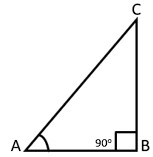In the above diagram Base = AB, Perpendicular = BC, Hypotenuse = AC and angle of reference = ∠A

## Trigonometrical Ratios

The ratio between the lengths of two sides of a right-angled triangle is known as trigonometrical ratios. There are six trigonometrical ratios present for a right-angled triangle, they are given below.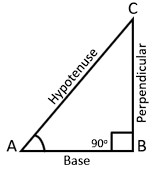Sin A = PerpendicularHypotenuse = BCAC

Cos A = BaseHypotenuse = ABAC

Tan A = PerpendicularBase = BCAB

Cot A = BasePerpendicular = ABBC

Sec A = HypotenuseBase = ACAB

Cosec A = HypotenusePerpendicular = ACBC

Each trigonometrical ratio is a real number and has no unit.

Example 1. From the below given figure find the value of Sin A, Cos A and Tan A.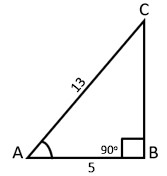Solution. As we can see, above figure is a wright angled triangle.

=> AC2 = AB2 + BC2

=> 132 = 52 + BC2

=> BC2 = 169 − 25

=> BC2 = 144

=> BC =  144

=> BC = 12

Sin A = BCAC = 1213

Cos A = ABAC = 513

Tan A = BCAB = 125

Example 2. In a right-angled triangle, if Tan A = 4/3 then find the value of Sin a, Cos A, Cot A, Sec A and Cosec A.

Solution.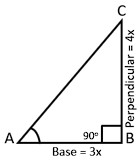Tan A = 43

=> Perpendicular/Base = 43

=> BCAB = 43

Let us assume AB = 3x and BC = 4x

AC2 = AB2 + BC2

=> AC2 = (3x)2 + (4x)2

=> AC2 = 9x2 + 16x2

=> AC =  25x2

=> AC = 5x

Sin A = 4x5x = 45

Cos A = 3x5x = 35

Cot A = 3x4x = 34

Cosec A = 5x4x = 54

Sec A = 5x3x = 53

Example 3. If Cos A = 4x5x, find the value of Tan A − Sec A.
Solution.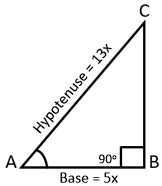Let's assume AB = 5x and AC = 13x.

AB2 + BC2 = AC2

=> BC2 = AC2 &miuns; AB2

=> BC2 = (13x)2 − (5x)2

=> BC2 = 169x2 − 25x2

=> BC =  144x2

=> BC = 12x

Tan A − Sec A

=> BCABACAB

=> 125135

=> (12−13)5

=> -15

Example 4. In the below given figure, ABC is a right-angled triangle. If BC = 5 cm and AC − BC = 1 cm, then find the value of Sin A and Sec A.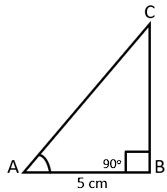Solution. Here AB = 5 cm and AC − BC = 1 cm.

AC = 1 + BC

As we know, in right angle triangle,

AC2 = AB2 + BC2

=> (1 + BC)2 = 25 + BC2

=> 1 + BC2 + 2BC = 25 + BC2

=> 2BC = 24

=> BC = 12 cm

AC − BC = 1

=> AC = 13 cm

Sin A = BCAC = 1213

Sec A = ACAB = 135

Example 5. In the below given triangle ABC, AD is perpendicular to BC. If BC = 112 cm, Tan B = 3/4 and Tan C = 5/12 . Find the length of BP and PC.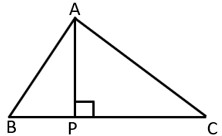Solution. As given Tan B = 34

=> APBP = 34

We can assume AP = 3x and BP = 4x

Similarly, Tan C = 512

=> APPC = 512

Here, we can assume AP = 5x and PC = 12x

BP + PC = 112

=> 4x + 12x = 112

=> 16x = 112

=> x = 11216

=> x = 7

BP = 4x = 4 × 7 = 28 cm

PC = 12x = 12 × 7 = 84 cm

## Reciprocal Relations

Sin A = PerpendicularHypotenuse and Cosec A = HypotenusePerpendicular

As we can see Sin A and Cosec A are reciprocal to each other.

Hence, Sin A = 1Cosec A and Cosec A = 1Sin A

Cos A = BaseHypotenuse and Sec A = HypotenuseBase

Hence, Cos A = 1sec A and Sec A = 1Cos A

Tan A = PerpendicularBase and Cot A = BasePerpendicular

Here, we can see Tan A and Cot A are reciprocal to each other.

Hence Tan A = 1Cot A and Cot A = 1Tan A

## Trigonometrical ratios of angles of 30o and 60o

In the below given diagram, lets assume ABC is an equilateral triangle having each side as 2a and AP is perpendicular to BC.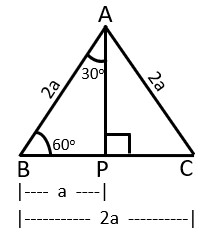Here BP = PC = a In right angle triangle AB2 = BP2 + AP2

=> AP2 = AB2 − BP2

=> AP2 = (2a)2 − a2

=> AP2 = 4a2 − a2

=> AP2 = 3a2

=> AP = 3a

Sin 60o = 3a2a = 32

Sin 30o = a2a = 12

Cos 60o = a2a = 12

Cos 30o = 3a2a = 32

Tan 60o = 3aa =  3

Tan 30o = a3a = 13

## Trigonometrical ratios of angles of 45o

Below given diagram is a right-angled isosceles triangle in which ∠B = 90o and AB = BC = a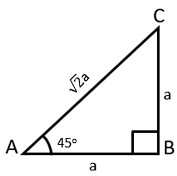Here ∠A = 45o

Sin 45o = a2a = 12

Cos 45o = a2a = 12

Tan 45o = aa = 1

Angle 0o 30o 45o 60o 90o
Sin 0 12 12 32 1
Cos 1 32 12 12 0
Tan 0 13 1  3  Not Defined
Cot Not Defined  3  1 13 0
Sec 1 23  2  2 Not Defined
Cosec Not Defined 2  2  23 1

From the above table, we can observe that as the angle increases from 0o to 90o

• Value of Sin increases from 0 to 1
• Value of Cos decreases from 1 to 0
• Value of Tan increases from 0 to infinite

Few formulas to remember for future problem solving.

1. Sin2A + Cos2A = 1
2. Sec2A − Tan2A = 1
3. Cosec2A − Cot2A = 1

Example 1. Find the value of Sin230o + 4 Cos290o + 5 Tan345o.

Solution. Sin230o + 4 Cos290o + 5 Tan345o

= (12)2 + 4 × (0)2 + 5 × (1)3

= 14 + 0 + 5

= 214

Example 2. If A = 45o, then verify Sec2A − Tan2A = 1.

Solution. Sec245o − Tan245o

= ( 2 )2 − (1)2

= 2 − 1

= 1

Example 3. If a = 15o, then find the value of 4 Sin 2a × Cos 3a × Cot 6a.

Solution. 4 Sin 2a × Cos 3a × Cot 6a

= 4 Sin 30o × Cos 45o × Cot 90o

= 4 × 12 × 12 × 0

= 0

Example 4. Prove that Sin 60o = 2 Tan 30o(1+Tan230o).

Solution. First consider LHS (Left Hand Side)

Sin 60o = 32

Now, lets consider RHS (Right Hand Side)

2 Tan 30o(1+Tan230o)

= 2 (131+13)

= 2343

= 23 × 34

=  3 2

Hence LHS = RHS.

## Class-9 Trigonometry MCQ

Trigonometry MCQ - 1

Trigonometry MCQ - 2

## Class-9 Trigonometry Worksheets

Trigonometry Worksheet - 1

Trigonometry Worksheet - 2

Trigonometry Worksheet - 3